# The implementation of the Norwegian Governmental Project Risk Assessment scheme

This entry is part 1 of 2 in the series The Norwegian Governmental Project Risk Assessment Scheme

### Introduction

In Norway all public investment projects with an expected budget exceeding NOK 750 million have to undergo quality assurance1 . The oil and gas sector, and state-owned companies with responsibility for their own investments, are exempt.

The quality assurance scheme2 consists of two parts: Quality assurance of the choice of concept – QA1 (Norwegian: KS1)3 and Quality assurance of the management base and cost estimates, including uncertainty analysis for the chosen project alternative – QA2 (Norwegian: KS2)4

This scheme is similar too many other countries’ efforts to create better cost estimates for public projects. One such example is Washington State Department of Transportations’ Cost Risk Assessment (CRA) and Cost Estimate Validation Process (CEVP®) (WSDOT, 2014).

One of the main purposes of QA2 is to set a cost frame for the project. This cost frame is to be approved by the government and is usually set to the 85% percentile (P85) of the estimated cost distribution. The cost frame for the responsible agency is usually set to the 50% percentile (P50). The difference between P50 and P85 is set aside as a contingency reserve for the project. This is reserves that ideally should remain unused.

The Norwegian TV program “Brennpunkt” an investigative program sponsored by the state television channel NRK put the light on the effects of this scheme5:

The investigation concluded that the Ministry of Finance quality assurance scheme had not resulted in reduced project cost overruns and that the process as such had been very costly.

This conclusion has of course been challenged.

The total cost for doing the risk assessments of the 85 projects was estimated to approx. NOK 400 million or more that \$60 million. In addition, in many cases, comes the cost of the quality assurance of choice of concept, a cost that probably is much higher.

### The Data

The data was assembled during the investigation and consists of six setts where five have information giving the P50 and P85 percentiles. The last set gives data on 29 projects finished before the QA2 regime was implemented (the data used in this article can be found as an XLSX.file here):

### The P85 and P50 percentiles

The first striking feature of the data is the close relation between the P85 and P50 percentiles: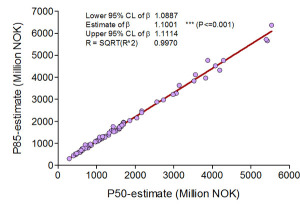In the graph above we have only used 83 of the 85 projects with known P50 and P85. The two that are omitted are large military projects. If they had been included, all the details in the graph had disappeared. We will treat these two projects separately later in the article.

A regression gives the relationship between P85 and P50 as:

P85 = (+/- 0.0113+1.1001)* P50, with R= 0.9970

The regression gives an exceptionally good fit. Even if the graph shows some projects deviating from the regression line, most falls on or close to the line.

With 83 projects this can’t be coincidental, even if the data represents a wide variety of government projects spanning from railway and roads to military hardware like tanks and missiles.

### The Project Cost Distribution

There is not much else to be inferred about the type of cost distribution from the graph. We do not know whether those percentiles came from fitted distributions or from estimated Pdf’s. This close relationship however leads us to believe that the individual projects cost distributions are taken from the same family of distributions.

If this family of distributions is a two-parameter distribution, we can use the known P50 and P856 percentiles to fit  a number of distributions to the data.

This use of quantiles to estimate the parameters of an a priori distribution have been described as “quantile maximum probability estimation” (Heathcote & al., 2004). This can be done by fitting a number of different a priori distributions and then compare the sum log likelihoods of the resulting best fits for each distribution, to find the “best” family of distributions.

Using this we anticipate finding cost distributions with the following properties:

1. Nonsymmetrical, with a short left and a long right tail i.e. being positive skewed and looking something like the distribution below (taken from a real life project):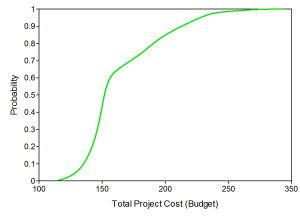2. The left tail we would expect to be short after the project has been run through the full QA1 and QA2 process. After two such encompassing processes we would believe that most, even if not all, possible avenues for cost reduction and grounds for miscalculations have been researched and exhausted – leaving little room for cost reduction by chance.

3. The right tail we would expect to be long taking into account the possibility of adverse price movements, implementation problems, adverse events etc. and thus the possibility of higher costs. This is where the project risk lies and where budget overruns are born.

4. The middle part should be quite steep indicating low volatility around “most probable cost”.

### Estimating the Projects Cost Distribution

To simplify we will assume that the above relation between P50 and P85 holds, and that it can be used to describe the resulting cost distribution from the projects QA2 risk assessment work.  We will hence use the P85/P50 ratio7  to study the cost distributions. This implies that we are looking for a family of distributions that have the probability of (X<1) =0.5 and the probability of (x<1.1) =0.85 and being positive skewed. This change of scale will not change the shape of the density function, but simply scale the graph horizontally.

Fortunately the MD Anderson Cancer Centre has a program – Parameter Solver8 – that can solve for the distribution parameters given the P50 and P85 percentiles (Cook, 2010). We can then use this to find the distributions that can replicate the P50 and P85 percentiles.

We find that distributions from the Normal, Log Normal, Gamma, Inverse Gamma and Weibull families will fit to the percentiles. All the distributions however are close to being symmetric with the exception of the Weibull distribution that has a left tail. A left tail in a budgeted cost distribution usually indicates over budgeting with the aim of looking good after the project has been finished. We do not think that this would have passed the QA2 process – so we don’t think that it has been used.

We believe that it is most likely that the distributions used are of the Normal, Gamma or of the Gamma derivative Erlang9 type, due to their convolution properties . That is, sums of independent identically distributed variables having one of these particular distributions come from the same distribution family. This makes it possible to simplify risk models of the cost only variety by just summing up the parameters10 of the cost elements to calculate the parameters of the total cost distribution.

This have the benefit of giving the closed form for the total cost distribution compared to Monte Carlo simulation where the closed form of the distribution, if it exists, only can be found thru the exercise we have done here.

This property can as well be a trap, as the adding up of cost items quickly gives the distribution of the sum symmetrical properties before it finally ends up as a Normal distribution11.

The figures in the graph below give the shapes for the Gamma and Normal distribution with the percentiles P50=1. and P85 = 1.1: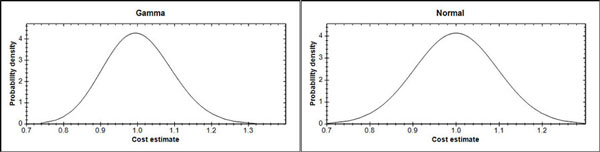The Normal distribution is symmetric and the Gamma distribution is also for all practical purposes symmetric. We therefore can conclude that the distributions for total project cost used in the 83 projects have been symmetric or close to symmetric distributions.
This result is quite baffling; it is difficult to understand why the project cost distributions should be symmetric.

The only economic explanation have to be that the expected cost of the projects are estimated with such precision that any positive or negative deviations are mere flukes and chance outside foreseeability and thus not included in the risk calculations.

But is this possible?

### The two Large Military Projects

The two projects omitted from the regression above: new fighter planes and frigates have values of the ratio P85/P50 as 1.19522 and 1.04543, compared to the regression estimate of 1.1001 for the 83 other projects. They are however not atypical, other among the 83 projects have both smaller (1.0310) and larger (1.3328) values for the P85/P50 ratio. Their sheer size however with a P85 of respective 68 and 18 milliard NOK, gives them a too high weight in a joint regression compared to the other projects.

Never the less, the same comments made above for the other 83 projects apply for these two projects. A regression with the projects included would have given the relationship between P85 and P50 as:

P85 = (+/- 0.0106+1.1751)* P50, with R= 0.9990.

And as shown in the graph below: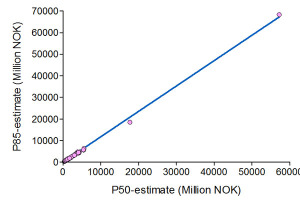This graph again depicts the surprisingly low variation in all the projects P85/P50 ratios:

The ratios have in point of fact a coefficient of variation of only 4.7% and a standard deviation of 0.052 – for the all the 85 projects.

### Conclusions

The Norwegian quality assurance scheme is obviously a large step in the direction of reduced budget overruns in public projects. (See: Public Works Projects)

Even if the final risk calculation somewhat misses the probable project cost distribution will the exercises described in the quality assurance scheme heighten both the risk awareness and the uncertainty knowingness. All, contributing to the common goal – reduced budget under- and overruns and reduced project cost.

It is nevertheless important that all elements in the quality assurance process catches the project uncertainties in a correct way, describing each projects specific uncertainty and its possible effects on project cost and implementation (See: Project Management under Uncertainty).

From what we have found: widespread use of symmetric cost distributions and possibly the same type of distributions across the projects, we are a little doubtful about the methods used for the risk calculations. The grounds for this are shown in the next two tables: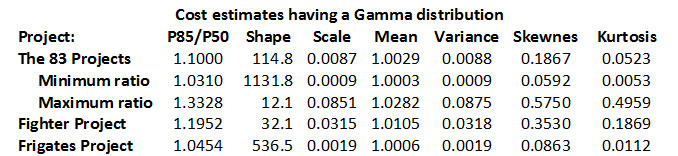The skewness12 given in the table above depends only on the shape parameter. The Gamma distribution will approach a normal distribution when the parameter larger than ten. In this case all projects’ cost distributions approach a normal distribution – that is a symmetric distribution with zero skewness.

To us, this indicates that the projects’ cost distribution reflects more the engineer’s normal calculation “errors” than the real risk for budget deviations due to implementation risk.

The kurtosis (excess kurtosis) indicates the form of the peak of the distribution. Normal distributions have zero kurtosis (mesocurtic) while distributions with a high peak have a positive kurtosis (leptokurtic).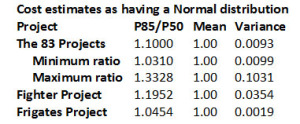It is stated in the QA2 that the uncertainty analysis shall have “special focus on … Event uncertainties represented by a binary probability distribution” If this part had been implemented we would have expected at least more flat-topped curves (platycurtic) with negative kurtosis or better not only unimodal distributions. It is hard to see traces of this in the material.

So, what can we so far deduct that the Norwegian government gets from the effort they spend on risk assessment of their projects?

First, since the cost distributions most probably are symmetric or near symmetric, expected cost will probably not differ significantly from the initial project cost estimate (the engineering estimate) adjusted for reserves and risk margins. We however need more data to substantiate this further.

Second, the P85 percentile could have been found by multiplying the P50 percentile by 1.1. Finding the probability distribution for the projects’ cost has for the purpose of establishing the P85 cost figures been unnecessary.

Third, the effect of event uncertainties seems to be missing.

Fourth, with such a variety of projects, it seems strange that the distributions for total project cost ends up being so similar. There have to be differences in project risk from building a road compared to a new Opera house.

Based on these findings it is pertinent to ask what went wrong in the implementation of QA2. The idea is sound, but the result is somewhat disappointing.

The reason for this can be that the risk calculations are done just by assigning probability distributions to the “aggregated and adjusted engineering “cost estimates and not by developing a proper simulation model for the project, taking into consideration uncertainties in all factors like quantities, prices, exchange rates, project implementation etc.

We will come back in a later post to the question if the risk assessment never the less reduces the budgets under- and overrun.

### References

Cook, John D. (2010), Determining distribution parameters from quantiles. http://www.johndcook.com/quantiles_parameters.pdf

Heathcote, A., Brown, S.& Cousineau, D. (2004). QMPE: estimating Lognormal, Wald, and Weibull RT distributions with a parameter-dependent lower bound. Journal of Behavior Research Methods, Instruments, and Computers (36), p. 277-290.

Washington State Department of Transportation (WSDOT), (2014), Project Risk Management Guide, Nov 2014. http://www.wsdot.wa.gov/projects/projectmgmt/riskassessment

### Endnotes

Series NavigationThe Estimated Project Cost Distributions and the Final Project Cost >>
1. The hospital sector has its own QA scheme. []
2. See, The Norwegian University of Science and Technology (NTNU): The Concept Research Programme []
3. The one page description for QA1 (Norwegian: KS1)have been taken from: NTNU’s Concept Research Programme []
4. The one page description for QA2 (Norwegian: KS2) have been taken from: NTNU’s Concept Research Programme []
5. The article also contains the data used here []
6. Most two-parameter families have sufficient flexibility to fit the P50 and P85 percentiles. []
7. If costs is normally distributed: C ∼ N (m, s2), then Z = C/m ∼ N (1, s2/ m2). If costs is gamma distributed: C ∼ Γ (a, λ) then Z = C/m ∼ Γ (1, λ). []